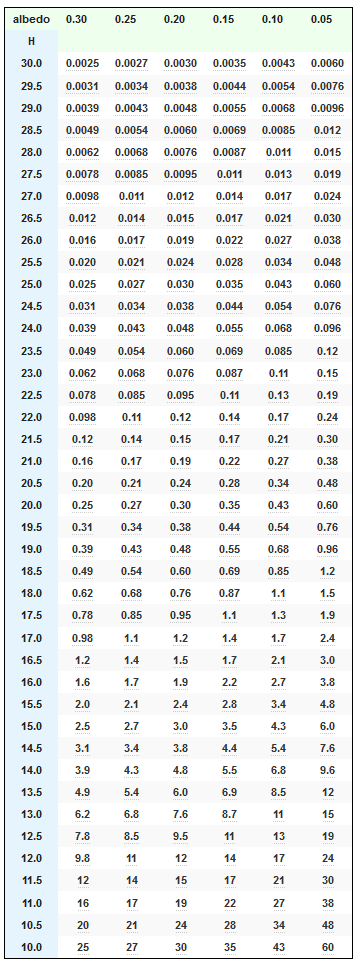# Estimating Asteroid Sizes Using Excel 2D Data Table

Quote of the Day

I do not give  performance guarantees because I provide the most flawed product known – people.

— Dave Neville, corporate recruiter

## Introduction

I recently gave a seminar on how to design products using Thermoelectric Coolers (TECs). During this presentation, I showed the audience how to generate various tables and graphs using Excel data tables. I was surprised to learn that no one in the audience had ever seen an Excel data table in action. Since the middle of a seminar on TECs is probably not the best time to divert to some Excel training, I decided to prepare a simpler example that would be easier to understand on first exposure.

My amateur astronomy activities soon provided me an excellent example. We often hear of Near-Earth Objects (NEOs) or asteroids  in the press (Figure 1). The sizes of these objects are estimated using a simple formula that is based on the objects brightness (i.e. absolute magnitude) and the percentage of incident light it reflects (i.e. albedo) – a perfect application for a data table.

Let's dig in …

## Background

You can skip the background material if you do not wish to know the details behind the formula used to create the data table.

### Scope

While crawling around the Jet Propulsion Laboratory web site on solar system objects, I found a table of asteroid sizes versus absolute visual magnitude and albedo. In this post, I will duplicate that JPL table using an Excel's data table, rounding to two significant digits,  and custom format features. This example provides a useful vehicle for illustrating how to use an Excel for a typical scientific application – the evaluation of a two-variable function.

### Definitions

The following definitions are repeated from this earlier blog post that was focused on using Mathcad.

Apparent Magnitude (V)
The visual brightness of an asteroid when observed and measured visually or with a CCD camera. (Source)
Absolute Magnitude (H)
The visual magnitude of an asteroid if it were 1 AU from the Earth and 1 AU from the Sun and fully illuminated, i.e. at zero phase angle – a geometrically impossible situation. (Source)
Geometric Albedo (pV)
The ratio of the brightness of a planetary body, as viewed from the Sun, to that of a white, diffusely reflecting sphere of the same size and at the same distance. Zero for a perfect absorber and 1 for a perfect reflector. (Source)
I smiled when I read this description of geometric albedo – it is VERY similar to the description of target strength as used by the sonar community. I am always amazed at the similarity between the various engineering and scientific disciplines.
Bond Albedo (A)
The Bond albedo is the fraction of power in the total electromagnetic radiation incident on an astronomical body that is scattered back out into space. The Bond albedo is related to the geometric albedo by the expression $latex A={{\rho }_{V}}\cdot q$, where q is termed the phase integral. We will not be worrying about the Bond albedo for this post. (Source)
Equivalent Spherical Diameter
The equivalent spherical diameter of an irregularly shaped object is the diameter of a sphere of equivalent volume. (Source)

### Asteroid Diameter Formula

JPL uses Equation 1 to estimate an asteroid's equivalent spherical diameter.

 Eq. 1 $latex \displaystyle D\left( {{{p}_{V}},H} \right)={{10}^{{0.5\cdot \left( {6.259-\text{log}\left( {{{p}_{V}}} \right)-0.4\cdot H} \right)}}}&s=2$

where

• D is the equivalent spherical diameter of the asteroid [km].
• pV is geometric albedo [dimensionless].
• H is absolute magnitude of the asteroid [dimensionless].

I have never seen Equation 1 before, however I have seen Equation 2 in a number of papers and websites (example), including the Minor Planet Center.

 Eq. 2 $latex \displaystyle D({{p}_{V}},H)=\frac{{1329}}{{\sqrt{{{{p}_{V}}}}}}\cdot {{10}^{{-0.2\cdot H}}}&s=2$

The detailed derivation of Equation 2 is a bit involved, but quite interesting. See this document for details (section 4.2).

Equation 1 appears to be different from Equation 2, but simple algebraic manipulation shows it to be almost identical – only a minor difference in the leading coefficient.

For my work here, I will use the JPL formula (Equation 1).

## Analysis

The actual workbook is included here for those who wish to see the details. If you want a step-by-step guide to making a data table, see this video by Danny Rocks – a bit old, but I have always thought Danny gave the best tutorials.

 Figure 2: Good Video Briefing on Spreadsheet Work.

The key points for my specific example (Figure 3) are:

• The data table function will substitute all the albedo values in into the row input cell.
• The data table function will substitute all the magnitude data (H) into the column input cell.
• The function output goes into cell C16. I placed a reference to cell C16 in cell B18, which the data table function uses to obtain the table values.
• I covered B18 with a couple of text boxes so I could label the row and column data.
• As JPL did, I rounded the data to preserve 2 significant figures.
• I used a custom number form ("[<1]0.####_0;[<10]0.0_0;0_0") to ensure the data table results were in the same format as used by JPL.

## Conclusion

I was able to duplicate JPL's table exactly using a data table, a custom format, and rounding to two significant digits.

## Appendix A: JPL Asteroid Diameter vs Mag. and Albedo

Here is the raw JPL table that I duplicated in Figure 3.Figure 4: JPL Asteroid Diameter vs Absolute Magnitude and Geometric Albedo.

This entry was posted in Astronomy, Excel. Bookmark the permalink.